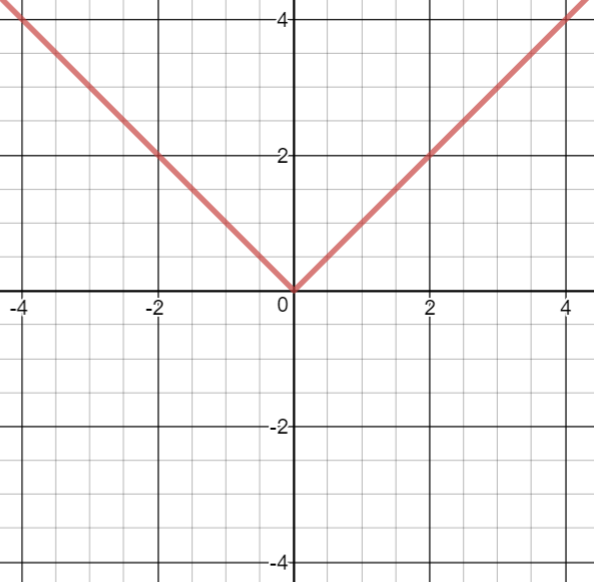# Limits and Continuity

## Objective

Define continuity of functions and determine whether a function is continuous on a particular domain.

## Criteria for Success

?

1. Define continuity of a function $f$ at a point $a$ as $f(a)$ exists, $\lim_{x\rightarrow a}f(x)$ exists, and $\lim_{x\rightarrow a}f(x)=f(a)$.
2. Determine whether a function meets the criteria for continuity on a particular domain.
3. Determine intervals on which a function is continuous.
4. Sketch functions given constraints on continuity.

## Tips for Teachers

?

The definition of continuity used in Anchor Problem #1 is a common definition, but the target task uses an alternate definition. Students should build an intuitive understanding of continuity and understand that there are multiple formal ways to describe this idea.

## Anchor Problems

?

### Problem 1

The conditions under which a function $f$  is "continuous" at a point $a$ are:

A: $f(a)$ exists

B: $\lim_{x\rightarrow a}f(x)$ exists

C: $\lim_{x\rightarrow a}f(x) = f(a)$

1. Sketch a function that meets all three conditions.
2. Sketch a function that meets conditions A and B but not C.
3. Sketch a function that meets condition A but not B or C.

### Problem 2

The graph below shows ${{f(x)}=|x|}$. Sketch a slope graph of ${f(x)}$.On what interval(s) is the slope graph continuous? What features of a function cause its slope graph to be discontinuous?

## Problem Set

?

The following resources include problems and activities aligned to the objective of the lesson that can be used to create your own problem set.

• Include problems generating graphs with different conditions for continuity and constraints from other lessons, including constraints given in interval notation
• Include practice evaluating limits, and use those limits to find whether a function is continuous
• Include practice determining on which domains a function is continuous, and writing those domains in interval notation
• Include problems analyzing slope graphs of different piecewise functions

Below are four conditions. If a point $a$ on a function $f$ meet these conditions, is it continuous?
A:  $\lim_{x\rightarrow a^+}f(x)$ exists
B:  $\lim_{x\rightarrow a^-}f(x)$ exists
C:  $f(a)$ exists
D:  $\lim_{x\rightarrow a^+}f(x)=\lim_{x\rightarrow a^-}f(x)=f(a)$